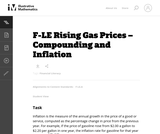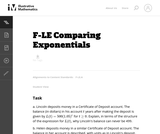# 4 Results

View
Selected filters:
• CCSS.Math.Content.HSF-LE.A.3Unrestricted Use
CC BY
Rating

The purpose of this task is to give students an opportunity to explore various aspects of exponential models (e.g., distinguishing between constant absolute growth and constant relative growth, solving equations using logarithms, applying compound interest formulas) in the context of a real world problem with ties to developing financial literacy skills.

Material Type:
Activity/Lab
Provider:
Illustrative Mathematics
Provider Set:
Illustrative Mathematics
Author:
Illustrative Mathematics
02/13/2013Only Sharing Permitted
CC BY-NC-ND
Rating

This lesson unit is intended to help teachers assess how well students are able to interpret exponential and linear functions and in particular to identify and help students who have the following difficulties: translating between descriptive, algebraic and tabular data, and graphical representation of the functions; recognizing how, and why, a quantity changes per unit intervale; and to achieve these goals students work on simple and compound interest problems.

Material Type:
Assessment
Lesson Plan
Provider:
Shell Center for Mathematical Education
U.C. Berkeley
Provider Set:
Mathematics Assessment Project (MAP)
04/26/2013Unrestricted Use
CC BY
Rating

This task gives students an opportunity to work with exponential functions in a real world context involving continuously compounded interest. They will study how the base of the exponential function impacts its growth rate and use logarithms to solve exponential equations.

Material Type:
Activity/Lab
Provider:
Illustrative Mathematics
Provider Set:
Illustrative Mathematics
Author:
Illustrative Mathematics
05/01/2012Only Sharing Permitted
CC BY-NC-ND
Rating

This lesson unit is intended to help teachers assess how well students are able to: articulate verbally the relationships between variables arising in everyday contexts; translate between everyday situations and sketch graphs of relationships between variables; interpret algebraic functions in terms of the contexts in which they arise; and reflect on the domains of everyday functions and in particular whether they should be discrete or continuous.

Material Type:
Assessment
Lesson Plan
Provider:
Shell Center for Mathematical Education
U.C. Berkeley
Provider Set:
Mathematics Assessment Project (MAP)